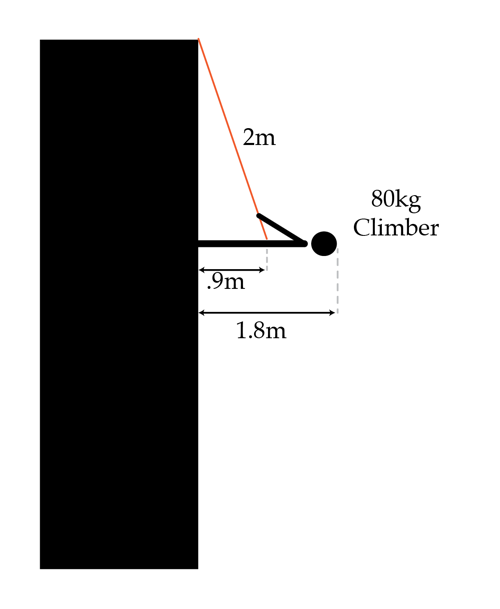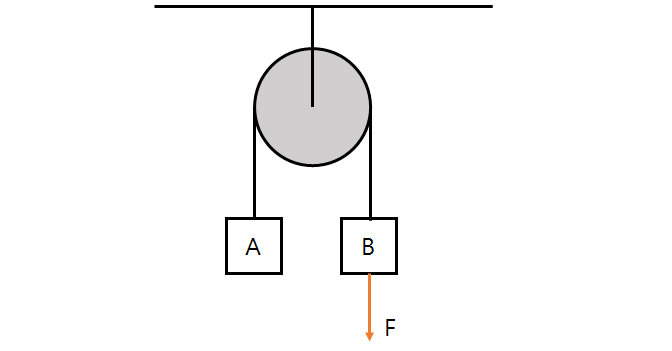Classical Mechanics

# Forces - Equilibrium

An object is being acted on by three forces. One force is in the positive direction with $5.5 \text{ N}$, and another is in the negative direction with $3.2 \text{ N}.$ What is the magnitude of the third force if the object has no acceleration?

An object of mass $610 \text{ g}$ is held on by a string that hangs from the ceiling. Two men pull the object downward with forces $1.7\text{ N}$ and $4.2\text{ N},$ respectively, but the object stays still. What is the approximate magnitude of tension in the string?

The acceleration of gravity on earth is about $9.8\text{ m/s}^2.$

A sandbag weighing $61.0 \text{ kg}$ hangs from a rope that is connected to the ceiling. If the sandbag is not moving, what is the approximate magnitude of the tension in the rope?

The acceleration of gravity on earth is about $9.81\text{ m/s}^2.$

A 80 kg climber is standing horizontally on a perfectly vertical cliff face as shown in the picture. The climber is 1.8 meters tall and is attached by a 2-meter-long rope fastened around the climber's middle to a point on the cliff above him. What is the normal force the cliff face exerts on the climber in Newtons?Details and assumptions

• The climber is not moving.
• The acceleration of gravity is $-9.8\text{ m/s}^2$.
• The center of mass of the climber is at the point where the rope meets their body.Two weights $A$ and $B$ weighing $7\text{ kg}$ and $3\text{ kg},$ respectively, are hanging on each end of a frictionless pulley fixed on the ceiling, as shown in the above diagram. As a force of $F$ is being applied to weight $B,$ the weights stay in equilibrium. Find the magnitude of $F.$

Assume that gravitational acceleration is $g=10\text{ m/s}^2.$

×